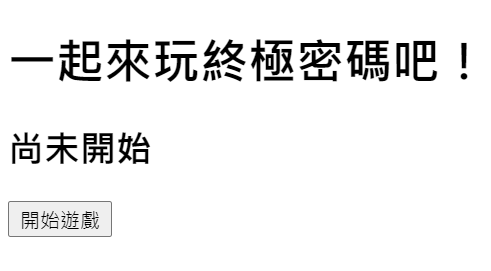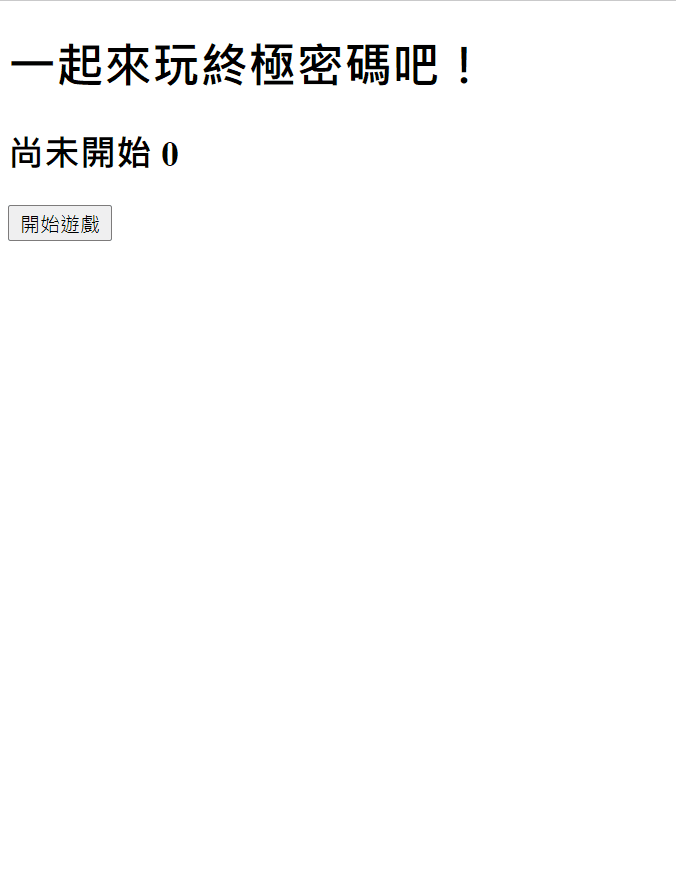#DAY 27
0

## 本日正文

### Hook - useEffect

(之前的寫法我們可能會將開始計時跟停止分別寫在 componentDidMount, componentWillUnmount 上)

### 前置作業 - 新增遊戲狀態

``````{status === "尚未開始" && <button onClick={startGame}>開始遊戲</button>}
{status === "進行中" ? (
<>
<hr />
<h3>
目前範圍：{guessRange} - {guessRange}
</h3>
<h4>請輸入所猜數字：{inputNum}</h4>
<input type="number" value={inputNum} onChange={handleInput} />
<button onClick={handleChange}>送出</button>
</>
): null }
{status === "恭喜答對！" && <button onClick={resetState}>重新遊戲</button>}
``````### 開始計時

``````const [count, setCount] = useState(0);
``````

``````useEffect(() => {
setInterval(() => setCount((c) => c + 1), 1000);
}, []);
````````````... (略)
const [start, setStart] = useState(false);
... (略)
useEffect(() => {
if (start) {
setInterval(() => setCount((c) => c + 1), 1000);
}
}, [start]);
``````

(PS. `[start]` 意思是當 start 有變化時才去執行這段)

startGame 裡面要做的事就是將 start 改為 true，還有 status 改為進行中，

``````const startGame = () => {
setStart(true);
setStatus("進行中");
};
````````````const id = setInterval(() => setCount((c) => c + 1), 1000);
return () => clearInterval(id);
``````

``````if (inputNum === answer) {
setStatus("恭喜答對！");
setInputNum("");
setStart(false);
return;
}
````````````setStart(false);
setCount(0);
``````